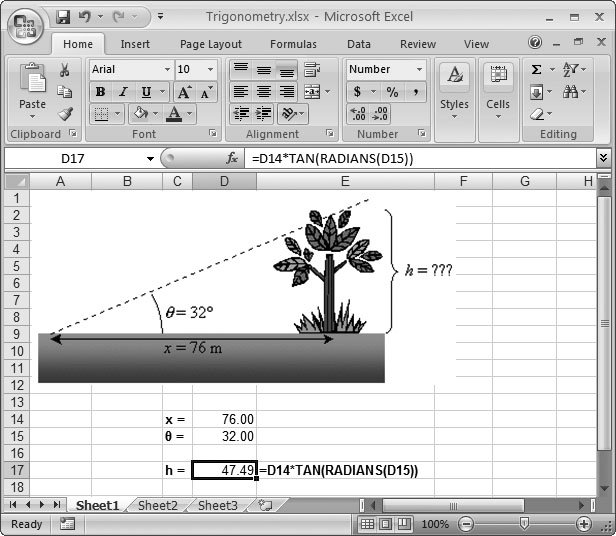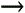Section 9.4. Trigonometry and Advanced Math

9.4. Trigonometry and Advanced Math

People sometimes describe trigonometry as the science of circles and angles. It's trigonometry that helps you calculate the hypotenuse of a triangle or the diameter of a circle. However, when you use trigonometry in Excel, you probably won't be worrying about shapes ; instead, you'll be using some type of formula from a scientific field that requires common trigonometric calculations like the cosine or tangent.

Students of the sciences know that trigonometry turns up anywhere you need to think about space, including geography, astronomy, kinematics, and optics. Less direct applications of trigonometry turn up in just about every other scientific fieldfrom chemistry to social science.

You can use Excel's trigonometric functions quite easily. Just choose the formula you need and the appropriate argument, much as you would use a pocket calculator. (Knowing when to use these trigonometry functions is another issue, and it may require a refresher course in mathematics.) Table 9-1 lists the functions you can use. Figure 9-11 shows how you can use trigonometry to solve a common high school math problem: you have the distance to a tree (which you can easily measure), and the angle between your current position and the top of the tree (which you can estimate). The problem is you have no way to judge the height of the tree.

Table 9-1. Trigonometry Functions

Function

Syntax

Description

ACOS( )

ACOS(number)

Calculates the inverse cosine (also known as the arccosine ) of a number.

ACOSH( )

ACOSH(number)

Calculates the inverse hyperbolic cosine of a number.

ASIN( )

ASIN(number)

Calculates the inverse sine (also known as the arcsine) of a number.

ATAN( )

ATAN(number)

Calculates the inverse tangent (also known as the arctangent) of a number.

ATANH( )

ATANH(number)

Calculates the inverse hyperbolic tangent of a number.

COS( )

COS(angle)

Calculates the cosine of an angle.

COSH( )

COSH(angle)

Calculates the hyperbolic cosine of an angle.

DEGREES( )

DEGREES(angle)

Converts an angle in radians into degrees. 360 degrees equals 2 radians.

EXP( )

EXP(power)

Calculates e (the natural logarithm constant), raised to the power you specify.

LN( )

LN(number)

Calculates the natural logarithm of a number (which is the logarithm with the base of e).

LOG( )

LOG(number, base)

Calculates the logarithm of a number with the base you specify.

LOG10( )

LOG10(number)

Calculates the base 10 logarithm of a number.

PI( )

PI( )

Returns the mathematical constant pi ( ), which is approximately 3.14.

Converts an angle in degrees into an angle in radians. 360 degrees equals 2 radians.

SIN( )

SIN(angle)

Calculates the sine of an angle.

SINH( )

SINH(angle)

Calculates the hyperbolic sine of an angle.

TAN( )

TAN(angle)

Calculates the tangent of an angle.

TANH( )

TANH(angle)

Calculates the hyperbolic tangent of an angle.Figure 9-11. You can solve this problem by using the trigonometric identity tan = h/x. You can rearrange this formula to find h (the height of the tree) so that h = x*tan. Be aware that Excel calculates the tangent using radians (radians are a measure of an angle, like degrees). Fortunately, Excel provides a RADIANS( ) function for converting degrees into radians, as well as a DEGREES( ) function for converting radians into degrees. In this example, the RADIANS( ) function was first used to convert degrees into radians; that result was then used as the argument in the TAN( ) function. The image was inserted using the InsertIllustrationsPicture command, which youll learn about in Chapter 19.

In addition to its trigonometric functions, Excel also includes a number of specialized mathematical functions, such as those used with matrices. These functions are summarized in Table 9-2.

Table 9-2. Miscellaneous Math Functions

Function

Syntax

Description

FACTDOUBLE( )

FACTDOUBLE(number)

Calculates the double factorial of a number. The double factorial's like a factorial, but it skips every second number. Thus, 5!! is 5*3*1 (whereas 5! is 5*4*3*2*1).

MDETERM( )

MDETERM(range)

Calculates the determinant of a matrix.

MINVERSE( )

MINVERSE(range)

Returns the inverse of a matrix. This function's an array function that returns multiple results.

MMULT( )

MMULT(range1, range2)

Returns the product of multiplying two matrixes . This function's an array function that returns multiple results.

MULTINOMIAL( )

MULTINOMIAL(range)

Returns the multinomial of a set of numbers . The multinomial's the ratio of the factorial of a sum of values to the product of factorials.

ROMAN ( )

ROMAN(number, [form])

A bizarre function that converts an ordinary number (from 1 to 3999) into Roman numerals. For example, ROMAN(4) returns the text IV . You may find large Roman numerals very confusing, so use the optional form argument to tell Excel to simplify the Roman numeral by applying a kind of rounding off effect. Form can range from 0 (which generates the unrounded Roman numeral) to 4 (which generates a much shorter but slightly less precise version). Search Excel's Help for ROMAN for a complete explanation.

SERIESSUM( )

SERIESSUM(x, n, m, coefficients)

Calculates the sum of a power series, where x is the base of the power series, n is the initial power to raise x to, m is the increment to the power for each term in the series, and co are the coefficients by which each successive power of x is multiplied. For more gory mathematical details, check out a detailed example of the series formula in Excel's function reference (choose FormulasFunction LibraryInsert Function, find the function, and click the "Help on this function link).

SQRTPI( )

SQRTPI(number)

Multiplies a number by pi ( ), and then calculates the square root of the result.Excel 2007[c] The Missing Manual
ISBN: 596527594
EAN: N/A
Year: 2007
Pages: 173